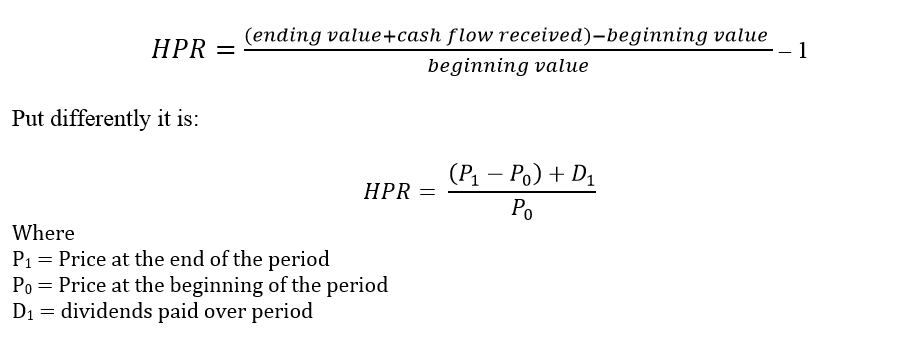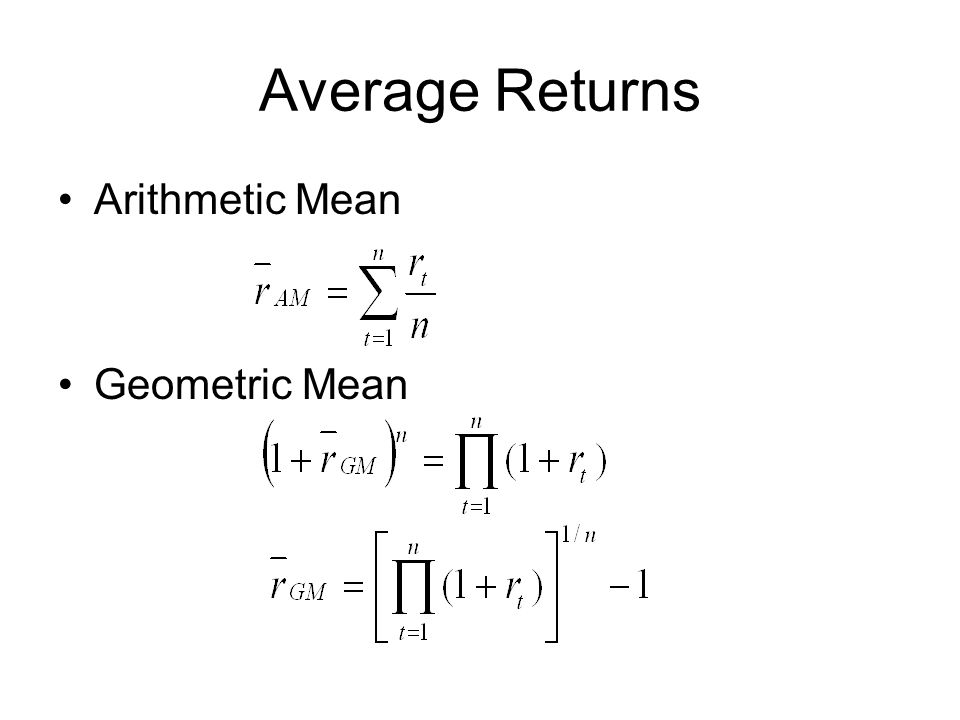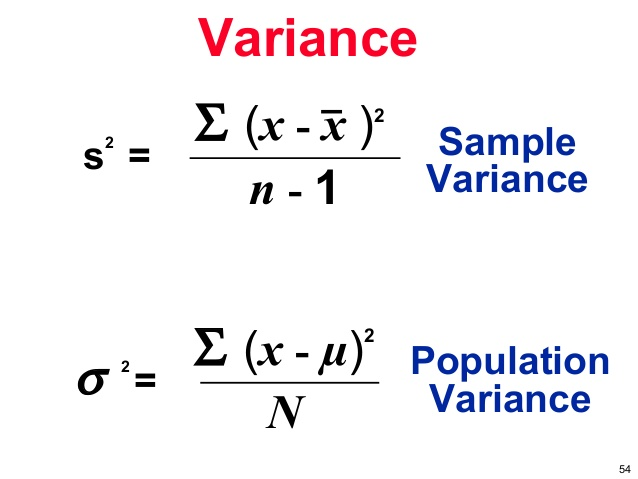# Reading 43: Portfolio Risk and Return (P.1)

1. Calculate and interpret major return measures and describe their appropriate uses
• Holding period return (HPR)• Average returns
• The arithmetic mean return is the simple average of a series of periodic returns.
• The geometric mean return is a compound annual rate.• The money-weighted rate of return is the internal rate of return on a portfolio based on all of its cash inflows and outflows.
• Other return measures
• Gross return: refers to the total return on a security portfolio before deducting fees for the management and administration of the investment account.
• Net return: refers to the return after these fees have been deducted.
• Pretax nominal return: return prior to paying taxes. Dividend income, interest income, short-term capital gains and long-term capital gains may be taxed at different rates.
• Real return: is nominal return adjusted for inflation.

Ex: Nominal return: 7%, inflation: 2%. ⇒ Approximately: 7-2 = 5%, real return: 1,07/1,02 = 4.9%

• A leveraged return: return to an investor that is a multiple of the return on the underlying asset. Equal (% of gains or losses on investment)/ cash investment.

2. Describe characteristics of the major asset that investors consider in forming portfolios

• Asset classes with the greatest average returns also have the highest standard deviations (risk) of returns.
• Liquidity is an additional characteristic to consider when choosing investments because liquidity effect the price and expected return of a securities.
• US, small-cap stocks ⇒ greatest average returns & greatest risk over period. Treasury bill ⇒ lowest (return & risk).

3. Calculate and interpret the mean, variance and covariance (correlation) of asset returns based on historical data.

• Variance (Standard deviation) of return for an Individual securityWhere: N (n): total number period

x, the mean or expected return

• Covariance and correlation of returns for two securities: Measures the extent to which 2 variables move together over time.
• Positive covariance ⇒ the variables (rates of return on 2 stocks) tend to move together.
• Negative covariance ⇒ 2 variables tend to move in opposite directions.
• Zero covariance ⇒ no linear relationship. Knowing 1 tells nothing about 2.
• Focus on the calculation of the covariance between 2 asset’s returns using Historical data.